Tags

There are 27 questions tagged under Equilibrium.

1

### Q:

A 100-newton weight is suspended by two cords as shown in the figure below. The tension in the slanted cord is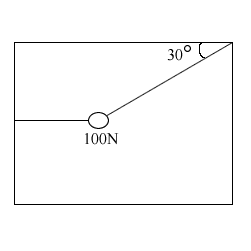200 N

100 N

50 N

### D

250 N

Tags: Forces | Equilibrium |

2

### Q:

If all the forces acting on an object balance so that the net force is zero, then

### A

the object's direction of motion can change, but not its speed

### B

the object's speed will decrease

### C

the object must be at rest

### D

none of the above

Tags: Forces | Equilibrium |

3

### Q:

After high salt intake, which of the following would you expect to happen to the blood?

### A

decreased blood pressure due to removal of salt from the blood and inflow to surrounding tissues

### B

decreased blood pressure due to an inflow of water to the blood from surrounding tissues

### C

increased blood pressure due to removal of salt from the blood and inflow to surrounding tissues

### D

increased blood pressure due to an inflow of water to the blood from surrounding tissues

Tags: Circulatory System | Equilibrium |

4

### Q:

In the endothermic reaction N2O4(g) <-->2NO2(g), what action can be taken in order to increase the equilibrium value of N2O4(g)?

### A

Increasing the volume of the container.

### B

Increasing the temperature of the system.

### C

Increasing the pressure of the system.

### D

Using a catalyst.

Tags: Equilibrium |

5

### Q:

A family sits on a 8m long see-saw. The daughter, massing 20kg, sits at the left end of the see-saw, and the father, massing 80kg, sits in the middle of the right portion of the see-saw. Where must the mother, massing 60kg, sit in order to make the see-saw in static equilibrium and level with the ground?

### A

1 m left of pivot

### B

1 m right of pivot

### C

4/3 m left of pivot

### D

4/3 m right of pivot

Tags: Equilibrium |

6

### Q:

At terminal velocity, an object has

### A

reached maximum elevation in its projectile arc with a vertical velocity of 0 m/s

### B

reached an equilibrium between the force of gravity and the drag force due to the air

### C

spent all of its potential energy

### D

reached maximum velocity and begun to decelerate

Tags: Forces | Equilibrium |

7

### Q:

Normally, in the process of osmosis, the net flow of water molecules into or out of the cell depends upon differences in the

### A

concentration of water molecules inside and outside the cell

### B

concentration of enzymes on either side of the cell membrane

### C

rate of molecular motion on either side of the cell membrane

### D

rate of movement of insoluble molecules inside the cell

Tags: Equilibrium |

8

### Q:

Two torques are applied on opposite ends of a pivot, counteracting one another. τ1 is applied using twice the force at half the distance of τ2; τ2 is applied at twice the distance of τ1 but half the force. Which of the following is true about the system?

### A

τ2 is causing an acceleration in its direction

### B

τ1 is causing an acceleration in its direction

### C

the system is in equilibrium

### D

the system experiences no motion

Tags: Equilibrium |

9

### Q:

Which of the following is true regarding torque?

### A

Its units are equivalent to Joules

### B

It does not need to be physically attached to its pivot

### C

It is only relevant to systems that possess an axle

### D

It can be calculated by taking the product of the force and the square of the distance from the pivot to the location where the force is applied

Tags: Equilibrium |

10

### Q: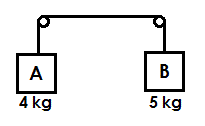In the schematic above, what is the magnitude of the downward acceleration of Block B?

0.9 m/s2

1.1 m/s2

5.5 m/s2

### D

10 m/s2

Tags: Forces | Equilibrium | Quantitative Skills |

11

### Q:

If a nonzero net torque is applied from an external source onto a frictionless rotating device, which of the following statements is false?

### A

The rotational velocity at some time t can be equal to zero after the torque begins.

### B

The final total rotational energy of the system can be greater than the total work done by the net torque.

### C

The total momentum of the device is constant before and after the torque is applied.

### D

Any gravitational potential energy gained by the device during rotation is lost upon completion of a full revolution.

Tags: Equilibrium |

12

### Q: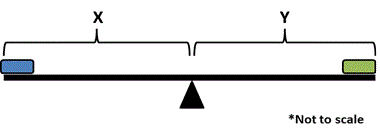A large board is held up by a pivot, as shown in the diagram above. It is known that 3X = Y. For the board to be in static equilibrium, which of the following must be true?

### A

The mass of the left block must be three times less than the mass of the right block

### B

The mass of the right block must be three times less than the mass of the left block

### C

The force of gravity on the left and right blocks are equal

### D

Net torque around the pivot need not be zero

Tags: Forces | Equilibrium | Quantitative Skills |

13

### Q:

Which of the following cannot be a coefficient for kinetic friction if we know that the coefficient of static friction is 0.4?

0

0.1

0.35

### D

0.45

Tags: Forces | Equilibrium |

14

### Q:

Which of the following is true in rotational equilibrium?

velocity is zero

### B

net torque is zero

### C

net force is zero

### D

angular momentum is zero

Tags: Equilibrium |

15

### Q: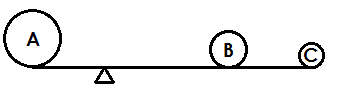A fulcrum with a lever is set up as in the diagram above. Ball A masses 150 kg and sits 80 cm from the pivot. Ball B masses 20 kg and sits 2 m from the pivot. If Ball C masses 5 kg, how far away from the pivot does it sit of the lever is in equilibrium?

1.6 m

8 m

16 m

### D

20 m

Tags: Forces | Equilibrium | Quantitative Skills |

16

### Q: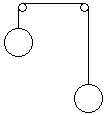The hanging objects in the system above have equal masses and are suspended by frictionless pulleys and massless strings. Which of the following best describes what will happen to the objects when released?

### A

The object on the left will accelerate downward until the object on the right hits the pulley.

### B

The object on the right will accelerate downward until the object on the left hits the pulley.

### C

The two objects will move to reach the same heights.

### D

The objects will not move.

Tags: Equilibrium |

17

### Q:

Which of the following correctly describes the point(s) that an object can oscillate around?

### A

Any equilibrium point

Any point

### C

Any stable equilibrium point

### D

Only certain stable equilibrium points

Tags: Equilibrium |

18

### Q:

A reaction with ΔH > 0 and ΔS > 0 will be:

nonspontaneous.

### B

always spontaneous.

### C

favorable at high temperatures.

### D

favorable at any temperature.

Tags: Equilibrium | Thermochemistry |

19

### Q:

Which of the following is a true statement regarding a perfectly balanced see-saw not in motion?

### A

There is no torque acting on the system.

### B

The system is in static equilibrium.

### C

There are no gravitational forces on the see-saw.

### D

The lengths of board on either side of the point of rotation must be equal.

Tags: Equilibrium |

20

### Q:

The equilibrium constant, K, and the reaction quotient, Q, for a reaction relate to each other in the sense that:

### A

K is equal to Q when the concentrations of reactants equal the concentrations of products.

### B

K can fluctuate however Q is constant despite reactant and product concentrations.

### C

Q is able to express a non-equilibrium state.

### D

Q reflects equilibrium at a specific temperature and pressure for a system.

Tags: Equilibrium |

21

### Q:

A rope is attached to a pulley from which an object of weight W is hung. Which of the following is the correct tension in the rope if we assume the object is suspended in the air in static equilibrium?

W

Wg

W/2

### D

Wg/2

Tags: Equilibrium |

22

### Q:

Which of the following correctly lists the equilibrium expression for 3A(s) --> 2B(aq) + C(l)?

[B]2[C]/[A]3

[B]2

[B]2[C]

### D

[B][C]/[A]

Tags: Equilibrium |

23

### Q:

Which of the following is true when the reaction quotient Q is less than the equilibrium constant K?

### A

The reaction is at equilibrium.

### B

The reaction will proceed to form more product.

### C

The reaction will reverse to form more reactant.

### D

The concentration of reactant is less than the concentration of product.

Tags: Equilibrium |

24

### Q:

Which of the following is FALSE of a chemical reaction in static equilibrium?

### A

Reactants are converted to products and products are converted to reactants at the same rate.

### B

Concentrations of reactants and products need not be equal.

### C

Concentrations of products is stable and unchanging.

### D

Products do not react with each other to form reactant.

Tags: Equilibrium |

25

### Q:

An exothermic reaction takes place in an enclosed chamber. The temperature of the chamber is decreased. The equilibrium of the reaction would be expected to:

shift left.

shift right.

remain the same.

### D

change however more data would be needed to determine whether the shift would be left or right.

Tags: Equilibrium |

26

### Q:

A large seesaw of length 25m is made where the fulcrum is a distance of 5m from the right end. A child of mass 25kg sits on the right end of the seesaw. A child of unknown mass sits on the left end of the seesaw. What is this child's mass?

5 kg

6.25 kg

7.25 kg

### D

7.75 kg

Tags: Equilibrium |

27

### Q:

A 55kg individual sits at the left end of a 2m seesaw and a 75kg individual sits on the right end. Where is the center of mass for this system?

### A

0.8 m from the left of the seesaw

### B

1 m from the left of the seesaw

### C

1.2 m from the left of the seesaw

### D

1.4 m from the left of the seesaw

Tags: Equilibrium |

Subscribe

Connect

Questions? We're here to help!